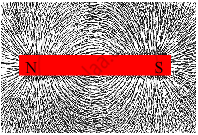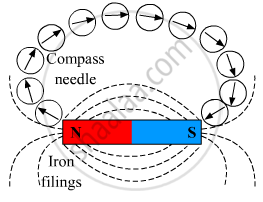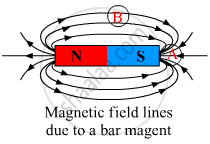# Explain with the Help of a Diagram How the Intensity and Direction of the Magnetic Field of a Bar Magnet Can Be Determined. - General Science

Write the answer to the following question:

Explain with the help of a diagram how the intensity and direction of the magnetic field of a bar magnet can be determined.

#### Solution

Take drawing cardboard and sprinkle some iron filings on it. Notice the position of the iron filings as a whole. Now, take a bar magnet and slowly bring it below the cardboard. You will observe that the iron filings tend to attract towards the magnet as shown below.To find the direction of the magnetic field around a bar magnet, place a magnetic compass with its South-Pole near one of the poles of the bar magnet. Locate and the point where the North-pole of the needle points. Now, lift the needle and place its South-Pole on the marked point. Now, again see where it North-Pole points. Repeat this process and you will get the direction of the magnetic field as shown below.To find the intensity of the magnetic field around a region of a bar magnet, we need to calculate the number of lines crossing through the unit area of that region. For example, if we have to calculate the intensity of the magnetic field at points A and B, then draw unit areas around A and B first and then calculate the number of lines passing through that area. By doing so, we see that the intensity of the field at A is greater than that at B.Concept: Magnetic Field
Is there an error in this question or solution?

#### APPEARS IN

Balbharati General Science 7th Standard Maharashtra State Board
Chapter 19 Properties of a Magnetic Field
Exercise | Q 3.5 | Page 130